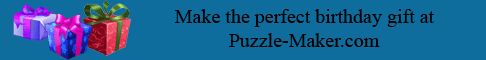Cliches 1--For when you are waiting   ``` 1. K N N L S S W X F Y B L E A S T M X S X F S E M X F K X C K Y X ``` ``` 2. L Y H M E O K A M X S X Y T M , K B W X Y T M C Y N N P Y N N E O K A M ``` ``` 3. S B M W K V Y C Y N N C K J M D O , K B W Y X C Y N N K N N P Y X X S L M X F M I ``` ``` 4. X F Y E Y E K O I S A M E E S P M N Y T Y B K X Y B L S O X Y S B E ``` ``` 5. X F M C K Y X Y B L Y E X F M F K I W M E X O K I X ``` ``` 6. E S T M W K V T V E F Y O C Y N N A S T M Y B ``` ``` 7. B S B M C E Y E L S S W B M C E ``` ```  ``` ```  ``` Copyright © 2012 Variety Games Inc.